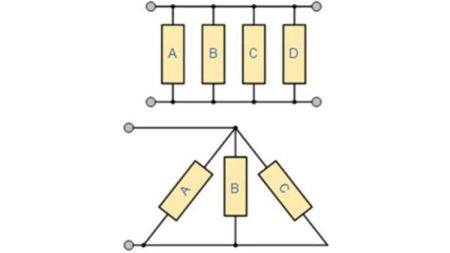﻿ Electronics S1W7: Resistors in Parallel » GFXhome

» » Electronics S1W7: Resistors in Parallel
Information of news
25-11-2020, 05:31

Electronics S1W7: Resistors in Parallel

Category: TutorialsMP4 | Video: h264, 1280x720 | Audio: AAC, 44.1 KHz, 2 Ch
Genre: eLearning | Language: English + .srt | Duration: 14 lectures (2h 25m) | Size: 633.2 MB

Students will learn how to solve parallel resistive circuits for voltage, current and resistance.

Prove that the total resistance in any parallel resistance circuit is smaller than the value of the smallest resistor.

Apply the Product Over the Sum Formula to detee the total resistance of any two resistors.

Apply Kirchhoff's Law and the Current Divider Formula to detee the individual branch currents in a parallel circuit.

Accurately solve parallel circuits for their resistances, voltages and currents.

Correctly detee the power for individual resistors and the total power in a parallel circuit.

No software, materials or supplies are required for this course.

A knowledge of series resistance rules and Ohm's Law are presumed. This information is covered in my course: Electronics S1W5: Resistors in Series.

During this course, the student will learn and apply the principles, laws and formulas that will lead to the solution of problems in parallelresistive circuits. By the end of this course, you will:

- Be able to state why the total resistance in any parallel resistance circuit is always smaller than the value of the smallest resistor in the circuit.

- Learn and demonstrate the use of the Parallel Resistance Formula to detee the resistance of parallel circuits.

- Apply the Product Over the Sum method to accurately detee the total resistance of any two parallel resistors.

- Learn and apply Kirchhoff's Current Law and the Current Divider Formula to detee the current flow in different branches of a parallel circuit.

- Learn how to correctly use the bar-bar symbol (||) to correctly write the formula for any parallel resistance circuit.

- State and apply the formula that will allow you to detee the total resistance of any number of resistors that have the same value.

- Solve seven parallel resistance problems presented by the instructor, using all of the rules and formulas you have learned to this point. The instructor will give you a detailed solution to each of the problems.

- Demonstrate that you can compute the total power in a circuit, and the individual power for each component.

Summary of Series and Parallel CircuitsThe course concludes with a thorough review of all of the formulas and laws of series and parallel resistance that you have learned so far.

This course presumes some knowledge of series resistive problems. These topics were covered in the prerequisite course: "Electronics S1W5:Resistors in Series". If you did not complete this course, you should be familiar with Ohm's Law and Kirchhoff's Voltage Law, so that you can solve series resistive circuits.

This course is composed of fourteen videos with a total rumnning of about 2.5 hours. Most videos run for 8 to 13 minutes, and each teaches one or more of the concepts shown in the bullet points above.

If you are headed for a career in electronics, or want to become a serious electronics hobbyist, or just want to know how electronics works, then this is a course for you.

If you are one of the following, this is an appropriate course for you:

An electronics professional who wants to review their skills.

Any individual desiring to pursue an electronics career.

A hobbyist who wants to know how electronic circuits work.

rapidgator

nitroflareSite BBcode/HTML Code:
Dear visitor, you went to the site as unregistered user.